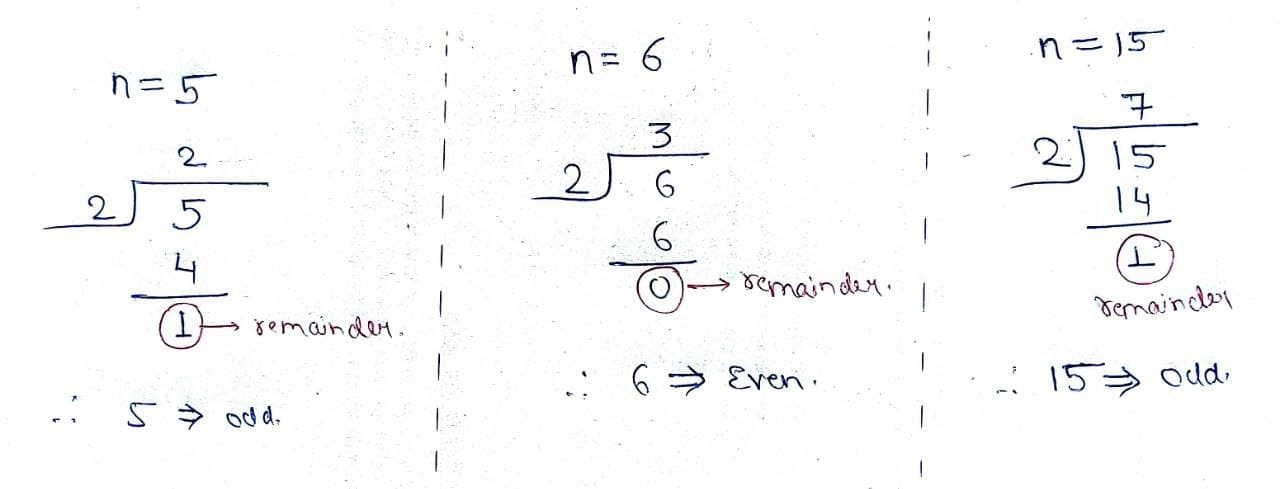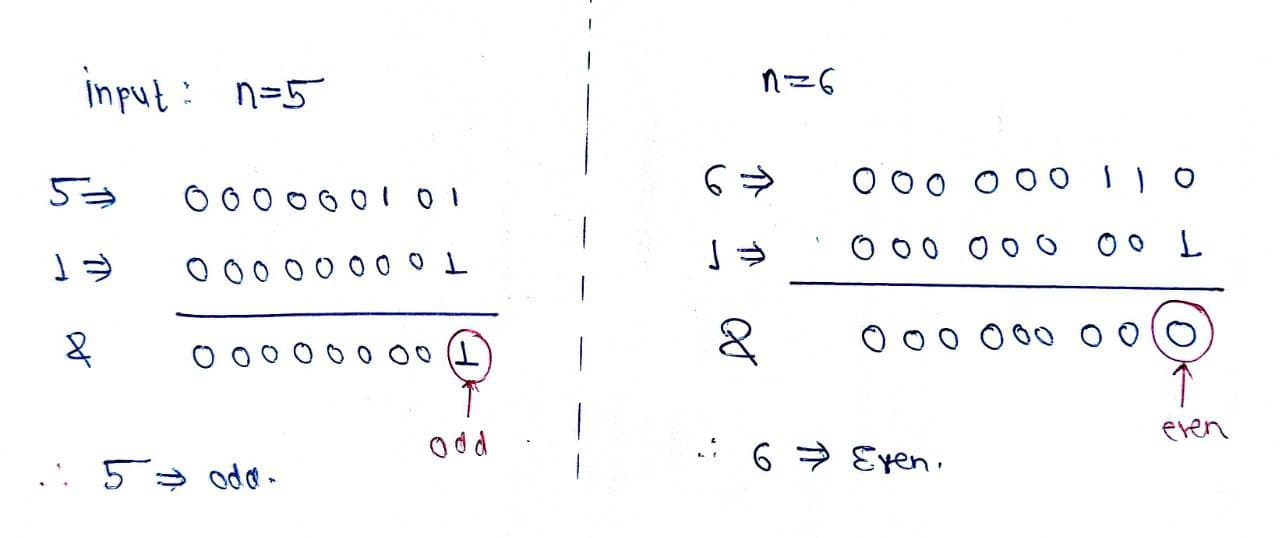# Check whether a given number is even or odd

Problem Statement: Given a number n, check whether a given number is even or odd.

Examples:

```Example 1:
Input: n=5
Output: odd
Explanation: 5 is not divisible by 2.

Example 2:
Input: n=6
Output: even
Explanation: 6 is divisible by 2.
```

### Solution

Disclaimer: Don’t jump directly to the solution, try it out yourself first.

Solution1: Using division

Intuition:  If we can prove that the given number is divisible by 2 then it will be even, otherwise it will be odd.

Approach:

• Find the remainder of the number.
• If it is 0 then the number is even otherwise it is odd.Code:

## C++ Code

``````#include<bits/stdc++.h>
using namespace std;

int remainder(int n) {
return (n % 2);
}

int main() {
int n = 5;
if (remainder(n) == 0) {
cout<<n <<" is even.";
} else {
cout<<n<<" is odd.";
}
}

``````

Output:

5 is odd.

Time complexity: O(1)

Space Complexity: O(1)

## Java Code

``````import java.util.*;

public class tuf {
public static void main(String[] args) {
int n = 5;
if (remainder(n) == 0) {
System.out.println(n + " is even.");
} else {
System.out.println(n + " is odd.");
}
}
public static int remainder(int n) {
return (n % 2);
}
}
``````

Output:

5 is odd.

Time complexity: O(1)

Space Complexity: O(1)

Solution2:  Using the bitwise operator

Intuition: As we know that bitwise operations are generally faster than normal operations so we should prefer it over normal operations.

Even Number: The last bit of even number is always 0.

Odd Number: Last bit of the odd number is always 1.

If we can get the last bit of any number then we can say that it is even or odd.

Approach:

• Take AND of n with 1.
• If it is 0 then print even otherwise odd.Code:

## C++ Code

``````#include<bits/stdc++.h>
using namespace std;
int solve(int n) {
if ((n & 1) == 0)
return 0;
else
return 1;
}

int main() {
int n = 5;
if (solve(n) == 0) {
cout<<n<<" is even.";
} else {
cout<<n << " is odd.";
}
}
``````

Output:

5 is odd.

Time complexity: O(1)

Space Complexity: O(1)

## Java Code

``````import java.util.*;
public class tuf {

public static void main(String[] args) {

int n = 5;
if (solve(n) == 0) {
System.out.println(n + " is even.");
} else {
System.out.println(n + " is odd.");
}
}
public static int solve(int n) {
if ((n & 1) == 0)
return 0;
else
return 1;
}
}
``````

Output:

5 is odd.

Time complexity: O(1)

Space Complexity: O(1)

Special thanks to Prashant Sahu for contributing to this article on takeUforward. If you also wish to share your knowledge with the takeUforward fam, please check out this article